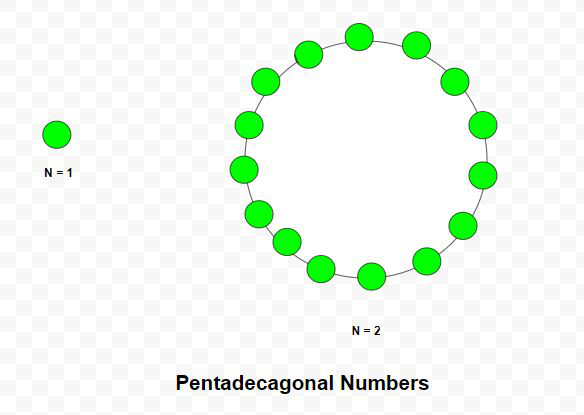Given a number N, the task is to find the Nth Pentadecagonal number.

A Pentadecagonal number is a figurate number that extends the concept of triangular and square numbers to the pentadecagon(a 15-sided polygon). The Nth pentadecagonal number counts the number of dots in a pattern of N nested pentadecagons, all sharing a common corner, where the ith tridecagon in the pattern has sides made of ‘i’ dots spaced one unit apart from each other. The first few Pentadecagonal numbers are 1, 15, 42, 82, 135, 201, 280 …

Examples:

Input: N = 2
Output: 15
Explanation:
The second Pentadecagonal number is 15.
Input: N = 6
Output: 201Approach: The Nth Pentadecagonal number is given by the formula:

``

Below is the implementation of the above approach:

## C++

 `// C++ program to find Nth` `// Pentadecagonal number` `#include ` `using` `namespace` `std;`   `// Function to find N-th` `// Pentadecagonal number` `int` `Pentadecagonal_num(``int` `n)` `{` `    ``// Formula to calculate nth` `    ``// Pentadecagonal number` `    ``return` `(13 * n * n - 11 * n) / 2;` `}`   `// Driver code` `int` `main()` `{` `    ``int` `n = 3;` `    ``cout << Pentadecagonal_num(n) << endl;` `   `  `    ``n = 10;` `    ``cout << Pentadecagonal_num(n) << endl;`   `    ``return` `0;` `}`

## Java

 `// Java program to find Nth` `// pentadecagonal number` `import` `java.io.*; ` `import` `java.util.*; `   `class` `GFG{ ` `    `  `// Function to find N-th` `// pentadecagonal number` `static` `int` `Pentadecagonal_num(``int` `n)` `{` `    `  `    ``// Formula to calculate nth` `    ``// Pentadecagonal number` `    ``return` `(``13` `* n * n - ``11` `* n) / ``2``;` `}` `    `  `// Driver code ` `public` `static` `void` `main(String[] args) ` `{ ` `    ``int` `n = ``3``;` `    ``System.out.println(Pentadecagonal_num(n));` `    `  `    ``n = ``10``;` `    ``System.out.println(Pentadecagonal_num(n));` `} ` `} `   `// This code is contributed by coder001`

## Python3

 `# Python3 program to find Nth ` `# pentadecagonal number `   `# Function to find N-th ` `# pentadecagonal number` `def` `Pentadecagonal_num(n):` `    `  `    ``# Formula to calculate nth ` `    ``# pentadecagonal number ` `    ``return` `(``13` `*` `n ``*` `n ``-` `11` `*` `n) ``/` `2`   `# Driver code    ` `n ``=` `3` `print``(``int``(Pentadecagonal_num(n))) `   `n ``=` `10` `print``(``int``(Pentadecagonal_num(n))) `   `# This code is contributed by divyeshrabadiya07 `

## C#

 `// C# program to find Nth ` `// pentadecagonal number ` `using` `System;` `class` `GFG{ ` `    `  `// Function to find N-th ` `// pentadecagonal number ` `static` `int` `Pentadecagonal_num(``int` `n) ` `{ ` `    `  `    ``// Formula to calculate nth ` `    ``// Pentadecagonal number ` `    ``return` `(13 * n * n - 11 * n) / 2; ` `} ` `    `  `// Driver code ` `public` `static` `void` `Main(``string``[] args) ` `{ ` `    ``int` `n = 3; ` `    ``Console.Write(Pentadecagonal_num(n) + ``"\n"``); ` `    `  `    ``n = 10; ` `    ``Console.Write(Pentadecagonal_num(n) + ``"\n"``); ` `} ` `} `   `// This code is contributed by rutvik_56`

## Javascript

 ``

Output:

```42
595```

Time complexity: O(1) as it is doing constant operations

Auxiliary space: O(1) as it is using constant space for variables

Feeling lost in the world of random DSA topics, wasting time without progress? It's time for a change! Join our DSA course, where we'll guide you on an exciting journey to master DSA efficiently and on schedule.
Ready to dive in? Explore our Free Demo Content and join our DSA course, trusted by over 100,000 geeks!

Previous
Next